### Learning Objectives

• Determine a new value of a quantity from the old value and the amount of change.
• Calculate the average rate of change and explain how it differs from the instantaneous rate of change.
• Apply rates of change to displacement, velocity, and acceleration of an object moving along a straight line.
• Predict the future population from the present value and the population growth rate.
• Use derivatives to calculate marginal cost and revenue in a business situation.

In this section we look at some applications of the derivative by focusing on the interpretation of the derivative as the rate of change of a function. These applications include acceleration and velocity in physics, population growth rates in biology, and marginal functions in economics.

# Amount of Change Formula

One application for derivatives is to estimate an unknown value of a function at a point by using a known value of a function at some given point together with its rate of change at the given point. If $f(x)$ is a function defined on an interval $[a,a+h]$, then the amount of change of $f(x)$ over the interval is the change in the $y$ values of the function over that interval and is given by

$f(a+h)-f(a)$.

The average rate of change of the function $f$ over that same interval is the ratio of the amount of change over that interval to the corresponding change in the $x$ values. It is given by

$\frac{f(a+h)-f(a)}{h}$.

As we already know, the instantaneous rate of change of $f(x)$ at $a$ is its derivative

$f^{\prime}(a)=\underset{h\to 0}{\lim}\frac{f(a+h)-f(a)}{h}$.

For small enough values of $h, \, f^{\prime}(a)\approx \frac{f(a+h)-f(a)}{h}$. We can then solve for $f(a+h)$ to get the amount of change formula:

$f(a+h)\approx f(a)+f^{\prime}(a)h$.

We can use this formula if we know only $f(a)$ and $f^{\prime}(a)$ and wish to estimate the value of $f(a+h)$. For example, we may use the current population of a city and the rate at which it is growing to estimate its population in the near future. As we can see in (Figure) , we are approximating $f(a+h)$ by the $y$ coordinate at $a+h$ on the line tangent to $f(x)$ at $x=a$. Observe that the accuracy of this estimate depends on the value of $h$ as well as the value of $f^{\prime}(a)$.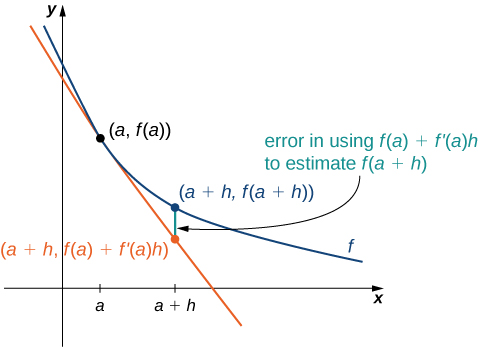Figure 1. The new value of a changed quantity equals the original value plus the rate of change times the interval of change: $f(a+h)\approx f(a)+f^{\prime}(a)h$.

Here is an interesting demonstration of rate of change.

### Estimating the Value of a Function

If $f(3)=2$ and $f^{\prime}(3)=5$, estimate $f(3.2)$.

#### Solution

Begin by finding $h$. We have $h=3.2-3=0.2$. Thus,

$f(3.2)=f(3+0.2)\approx f(3)+(0.2)f^{\prime}(3)=2+0.2(5)=3$.

Given $f(10)=-5$ and $f^{\prime}(10)=6$, estimate $f(10.1)$.

#### Hint

Use the same process as in the preceding example.

-4.4

# Motion along a Line

Another use for the derivative is to analyze motion along a line. We have described velocity as the rate of change of position. If we take the derivative of the velocity, we can find the acceleration , or the rate of change of velocity. It is also important to introduce the idea of speed , which is the magnitude of velocity. Thus, we can state the following mathematical definitions.

### Definition

Let $s(t)$ be a function giving the position of an object at time $t$.

The velocity of the object at time $t$ is given by $v(t)=s^{\prime}(t)$.

The speed of the object at time $t$ is given by $|v(t)|$.

The acceleration of the object at $t$ is given by $a(t)=v^{\prime}(t)=s''(t)$.

### Comparing Instantaneous Velocity and Average Velocity

A ball is dropped from a height of 64 feet. Its height above ground (in feet) $t$ seconds later is given by $s(t)=-16t^2+64$.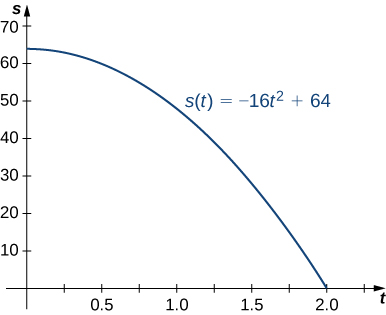1. What is the instantaneous velocity of the ball when it hits the ground?
2. What is the average velocity during its fall?

#### Solution

The first thing to do is determine how long it takes the ball to reach the ground. To do this, set $s(t)=0$. Solving $-16t^2+64=0$, we get $t=2$, so it takes 2 seconds for the ball to reach the ground.

1. The instantaneous velocity of the ball as it strikes the ground is $v(2)$. Since $v(t)=s^{\prime}(t)=-32t$ m we obtain $v(t)=-64$ ft/s.
2. The average velocity of the ball during its fall is
$v_{avg}=\frac{s(2)-s(0)}{2-0}=\frac{0-64}{2}=-32$ ft/s.

### Interpreting the Relationship between $v(t)$ and $a(t)$

A particle moves along a coordinate axis in the positive direction to the right. Its position at time $t$ is given by $s(t)=t^3-4t+2$. Find $v(1)$ and $a(1)$ and use these values to answer the following questions.

1. Is the particle moving from left to right or from right to left at time $t=1$?
2. Is the particle speeding up or slowing down at time $t=1$?

#### Solution

Begin by finding $v(t)$ and $a(t)$.

$v(t)=s^{\prime}(t)=3t^2-4$ and $a(t)=v^{\prime}(t)=s''(t)=6t$.

Evaluating these functions at $t=1$, we obtain $v(1)=-1$ and $a(1)=6$.

1. Because $v(1) < 0$, the particle is moving from right to left.
2. Because $v(1) < 0$ and $a(1) < 0$, velocity and acceleration are acting in opposite directions. In other words, the particle is being accelerated in the direction opposite the direction in which it is traveling, causing $|v(t)|$ to decrease. The particle is slowing down.

### Position and Velocity

The position of a particle moving along a coordinate axis is given by $s(t)=t^3-9t^2+24t+4, \, t\ge 0$.

1. Find $v(t)$.
2. At what time(s) is the particle at rest?
3. On what time intervals is the particle moving from left to right? From right to left?
4. Use the information obtained to sketch the path of the particle along a coordinate axis.

#### Solution

1. The velocity is the derivative of the position function:
$v(t)=s^{\prime}(t)=3t^2-18t+24$.
2. The particle is at rest when $v(t)=0$, so set $3t^2-18t+24=0$. Factoring the left-hand side of the equation produces $3(t-2)(t-4)=0$. Solving, we find that the particle is at rest at $t=2$ and $t=4$.
3. The particle is moving from left to right when $v(t) < 0$ and from right to left when $v(t) < 0$.  (Figure) gives the analysis of the sign of $v(t)$ for $t\ge 0$, but it does not represent the axis along which the particle is moving.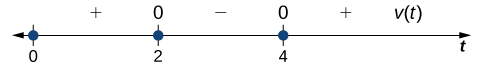The sign of v(t) determines the direction of the particle.

Since $3t^2-18t+24 < 0$ on $[0,2)\cup (2,+\infty)$, the particle is moving from left to right on these intervals. Since $3t^2-18t+24 < 0$ on $(2,4)$, the particle is moving from right to left on this interval.

4. Before we can sketch the graph of the particle, we need to know its position at the time it starts moving $(t=0)$ and at the times that it changes direction $(t=2,4)$. We have $s(0)=4, \, s(2)=24$, and $s(4)=20$. This means that the particle begins on the coordinate axis at 4 and changes direction at 0 and 20 on the coordinate axis. The path of the particle is shown on a coordinate axis in (Figure) .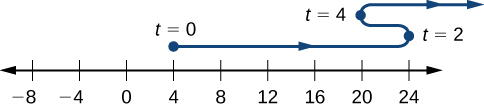The path of the particle can be determined by analyzing $v(t)$.

A particle moves along a coordinate axis. Its position at time $t$ is given by $s(t)=t^2-5t+1$. Is the particle moving from right to left or from left to right at time $t=3$?

#### Hint

Find $v(3)$ and look at the sign.

left to right

# Population Change

In addition to analyzing velocity, speed, acceleration, and position, we can use derivatives to analyze various types of populations, including those as diverse as bacteria colonies and cities. We can use a current population, together with a growth rate, to estimate the size of a population in the future. The population growth rate is the rate of change of a population and consequently can be represented by the derivative of the size of the population.

### Definition

If $P(t)$ is the number of entities present in a population, then the population growth rate of $P(t)$ is defined to be $P^{\prime}(t)$.

### Estimating a Population

The population of a city is tripling every 5 years. If its current population is 10,000, what will be its approximate population 2 years from now?

#### Solution

Let $P(t)$ be the population (in thousands) $t$ years from now. Thus, we know that $P(0)=10$ and based on the information, we anticipate $P(5)=30$. Now estimate $P^{\prime}(0)$, the current growth rate, using

$P^{\prime}(0)\approx \frac{P(5)-P(0)}{5-0}=\frac{30-10}{5}=4$.

By applying (Figure) to $P(t)$, we can estimate the population 2 years from now by writing

$P(2)\approx P(0)+(2)P^{\prime}(0)\approx 10+2(4)=18$;

thus, in 2 years the population will be approximately 18,000.

The current population of a mosquito colony is known to be 3,000; that is, $P(0)=3,000$. If $P^{\prime}(0)=100$, estimate the size of the population in 3 days, where $t$ is measured in days.

#### Hint

Use $P(3)\approx P(0)+3P^{\prime}(0)$.

3,300

# Changes in Cost and Revenue

In addition to analyzing motion along a line and population growth, derivatives are useful in analyzing changes in cost, revenue, and profit. The concept of a marginal function is common in the fields of business and economics and implies the use of derivatives. The marginal cost is the derivative of the cost function. The marginal revenue is the derivative of the revenue function. The marginal profit i s the derivative of the profit function, which is based on the cost function and the revenue function.

### Definition

If $C(x)$ is the cost of producing $x$ items, then the marginal cost $MC(x)$ is $MC(x)=C^{\prime}(x)$.

If $R(x)$ is the revenue obtained from selling $x$ items, then the marginal revenue $MR(x)$ is $MR(x)=R^{\prime}(x)$.

If $P(x)=R(x)-C(x)$ is the profit obtained from selling $x$ items, then the marginal profit $MP(x)$ is defined to be $MP(x)=P^{\prime}(x)=MR(x)-MC(x)=R^{\prime}(x)-C^{\prime}(x)$.

We can roughly approximate

$MC(x)=C^{\prime}(x)=\underset{h\to 0}{\lim}\frac{C(x+h)-C(x)}{h}$

by choosing an appropriate value for $h$. Since $x$ represents objects, a reasonable and small value for $h$ is 1. Thus, by substituting $h=1$, we get the approximation $MC(x)=C^{\prime}(x)\approx C(x+1)-C(x)$. Consequently, $C^{\prime}(x)$ for a given value of $x$ can be thought of as the change in cost associated with producing one additional item. In a similar way, $MR(x)=R^{\prime}(x)$ approximates the revenue obtained by selling one additional item, and $MP(x)=P^{\prime}(x)$ approximates the profit obtained by producing and selling one additional item.

### Applying Marginal Revenue

Assume that the number of barbeque dinners that can be sold, $x$, can be related to the price charged, $p$, by the equation $p(x)=9-0.03x, \, 0\le x\le 300$.

In this case, the revenue in dollars obtained by selling $x$ barbeque dinners is given by

$R(x)=xp(x)=x(9-0.03x)=-0.03x^2+9x$ for $0\le x\le 300$.

Use the marginal revenue function to estimate the revenue obtained from selling the 101st barbeque dinner. Compare this to the actual revenue obtained from the sale of this dinner.

#### Solution

First, find the marginal revenue function: $MR(x)=R^{\prime}(x)=-0.06x+9$.

### Key Concepts

• Using $f(a+h)\approx f(a)+f^{\prime}(a)h$, it is possible to estimate $f(a+h)$ given $f^{\prime}(a)$ and $f(a)$.
• The rate of change of position is velocity, and the rate of change of velocity is acceleration. Speed is the absolute value, or magnitude, of velocity.
• The population growth rate and the present population can be used to predict the size of a future population.
• Marginal cost, marginal revenue, and marginal profit functions can be used to predict, respectively, the cost of producing one more item, the revenue obtained by selling one more item, and the profit obtained by producing and selling one more item.

For the following exercises, the given functions represent the position of a particle traveling along a horizontal line.

1. Find the velocity and acceleration functions.
2. Determine the time intervals when the object is slowing down or speeding up.

1.  $s(t)=2t^3-3t^2-12t+8$

2.  $s(t)=2t^3-15t^2+36t-10$

#### Solution

a. $v(t)=6t^2-30t+36, \, a(t)=12t-30$
b. Speeds up: $(2,2.5)\cup (3,\infty)$; Slows down: $(0,2)\cup (2.5,3)$

3.  $s(t)=\frac{t}{1+t^2}$

4.  A rocket is fired vertically upward from the ground. The distance $s$ in feet that the rocket travels from the ground after $t$ seconds is given by $s(t)=-16t^2+560t$.

1. Find the velocity of the rocket 3 seconds after being fired.
2. Find the acceleration of the rocket 3 seconds after being fired.

#### Solution

a. $464 \, \text{ft/s}^2$
b. $-32 \, \text{ft/s}^2$

5.  A ball is thrown downward with a speed of 8 ft/s from the top of a 64-foot-tall building. After $t$ seconds, its height above the ground is given by $s(t)=-16t^2-8t+64$.

1. Determine how long it takes for the ball to hit the ground.
2. Determine the velocity of the ball when it hits the ground.

6.  The position function $s(t)=t^2-3t-4$ represents the position of the back of a car backing out of a driveway and then driving in a straight line, where $s$ is in feet and $t$ is in seconds. In this case, $s(t)=0$ represents the time at which the back of the car is at the garage door, so $s(0)=-4$ is the starting position of the car, 4 feet inside the garage.

1. Determine the velocity of the car when $s(t)=0$.
2. Determine the velocity of the car when $s(t)=14$.
a. 5 ft/s b. 9 ft/s

7.  The position of a hummingbird flying along a straight line in $t$ seconds is given by $s(t)=3t^3-7t$ meters.

1. Determine the velocity of the bird at $t=1$ sec.
2. Determine the acceleration of the bird at $t=1$ sec.
3. Determine the acceleration of the bird when the velocity equals 0.

8.  A potato is launched vertically upward with an initial velocity of 100 ft/s from a potato gun at the top of an 85-foot-tall building. The distance in feet that the potato travels from the ground after $t$ seconds is given by $s(t)=-16t^2+100t+85$.

1. Find the velocity of the potato after 0.5 sec and 5.75 sec.
2. Find the speed of the potato at 0.5 sec and 5.75 sec.
3. Determine when the potato reaches its maximum height.
4. Find the acceleration of the potato at 0.5 s and 1.5 s.
5. Determine how long the potato is in the air.
6. Determine the velocity of the potato upon hitting the ground.

#### Solution

a. 84 ft/s, -84 ft/s
b. 84 ft/s
c. $\frac{25}{8}$ sec
d. $-32 \, \text{ft/s}^2$ in both cases
e. $\frac{1}{8}(25+\sqrt{965})$ sec
f. $-4\sqrt{965}$ ft/s

9.  The position function $s(t)=t^3-8t$ gives the position in miles of a freight train where east is the positive direction and $t$ is measured in hours.

1. Determine the direction the train is traveling when $s(t)=0$.
2. Determine the direction the train is traveling when $a(t)=0$.
3. Determine the time intervals when the train is slowing down or speeding up.

10.  The following graph shows the position $y=s(t)$ of an object moving along a straight line.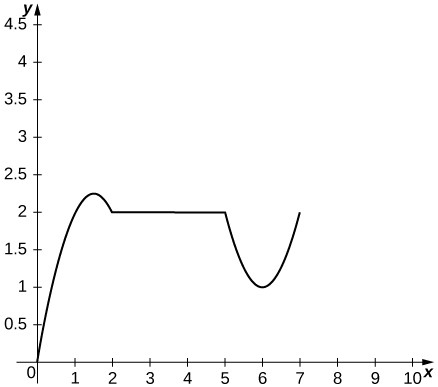1. Use the graph of the position function to determine the time intervals when the velocity is positive, negative, or zero.
2. Sketch the graph of the velocity function.
3. Use the graph of the velocity function to determine the time intervals when the acceleration is positive, negative, or zero.
4. Determine the time intervals when the object is speeding up or slowing down.

#### Solution

a. Velocity is positive on $(0,1.5)\cup (6,7)$, negative on $(1.5,2)\cup (5,6)$, and zero on $(2,5)$.
b.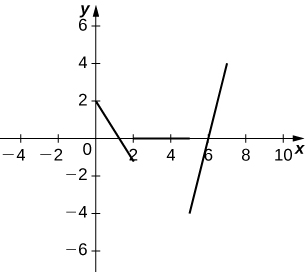c. Acceleration is positive on $(5,7)$, negative on $(0,2)$, and zero on $(2,5)$.
d. The object is speeding up on $(6,7)\cup (1.5,2)$ and slowing down on $(0,1.5)\cup (5,6)$.

11.  The cost function, in dollars, of a company that manufactures food processors is given by $C(x)=200+\frac{7}{x}+\frac{x^2}{7}$, where $x$ is the number of food processors manufactured.

1. Find the marginal cost function.
2. Find the marginal cost of manufacturing 12 food processors.
3. Find the actual cost of manufacturing the thirteenth food processor.

12.  The price $p$ (in dollars) and the demand $x$ for a certain digital clock radio is given by the price-demand function $p=10-0.001x$.

1. Find the revenue function $R(x)$.
2. Find the marginal revenue function.
3. Find the marginal revenue at $x=2000$ and $x=5000$.

#### Solution

a. $R(x)=10x-0.001x^2$
b. $R^{\prime}(x)=10-0.002x$
c. $6 per item,$0 per item

13. [T] A profit is earned when revenue exceeds cost. Suppose the profit function for a skateboard manufacturer is given by $P(x)=30x-0.3x^2-250$, where $x$ is the number of skateboards sold.

1. Find the exact profit from the sale of the thirtieth skateboard.
2. Find the marginal profit function and use it to estimate the profit from the sale of the thirtieth skateboard.

14. [T] In general, the profit function is the difference between the revenue and cost functions: $P(x)=R(x)-C(x)$.

Suppose the price-demand and cost functions for the production of cordless drills is given respectively by $p=143-0.03x$ and $C(x)=75,000+65x$, where $x$ is the number of cordless drills that are sold at a price of $p$ dollars per drill and $C(x)$ is the cost of producing $x$ cordless drills.

1. Find the marginal cost function.
2. Find the revenue and marginal revenue functions.
3. Find $R^{\prime}(1000)$ and $R^{\prime}(4000)$. Interpret the results.
4. Find the profit and marginal profit functions.
5. Find $P^{\prime}(1000)$ and $P^{\prime}(4000)$. Interpret the results.

#### Solution

a. $C^{\prime}(x)=65$
b. $R(x)=143x-0.03x^2, \, R^{\prime}(x)=143-0.06x$
c. $R^{\prime}(1000)=83, \, R^{\prime}(4000)=-97$. At a production level of 1000 cordless drills, revenue is increasing at a rate of $83 per drill; at a production level of 4000 cordless drills, revenue is decreasing at a rate of$97 per drill.
d. $P(x)=-0.03x^2+78x-75000, \, P^{\prime}(x)=-0.06x+78$
e. $P^{\prime}(1000)=18, \, P^{\prime}(4000)=-162$. At a production level of 1000 cordless drills, profit is increasing at a rate of $18 per drill; at a production level of 4000 cordless drills, profit is decreasing at a rate of$162 per drill.

15.  A small town in Ohio commissioned an actuarial firm to conduct a study that modeled the rate of change of the town’s population. The study found that the town’s population (measured in thousands of people) can be modeled by the function $P(t)=-\frac{1}{3}t^3+64t+3000$, where $t$ is measured in years.

1. Find the rate of change function $P^{\prime}(t)$ of the population function.
2. Find $P^{\prime}(1), \, P^{\prime}(2), \, P^{\prime}(3)$, and $P^{\prime}(4)$. Interpret what the results mean for the town.
3. Find $P''(1), \, P''(2), \, P''(3)$, and $P''(4)$. Interpret what the results mean for the town’s population.

16. [T] A culture of bacteria grows in number according to the function $N(t)=3000(1+\frac{4t}{t^2+100})$, where $t$ is measured in hours.

1. Find the rate of change of the number of bacteria.
2. Find $N^{\prime}(0), \, N^{\prime}(10), \, N^{\prime}(20)$, and $N^{\prime}(30)$.
3. Interpret the results in (b).
4. Find $N''(0), \, N''(10), \, N''(20)$, and $N''(30)$. Interpret what the answers imply about the bacteria population growth.

#### Solution

a. $N^{\prime}(t)=3000(\frac{-4t^2+400}{(t^2+100)^2})$
b. $N^{\prime}(0)=120, \, N^{\prime}(10)=0, \, N^{\prime}(20)=-14.4, \, N^{\prime}(30)=-9.6$
c. The bacteria population increases from time 0 to 10 hours; afterwards, the bacteria population decreases.
d. $N''(0)=0, \, N''(10)=-6, \, N''(20)=0.384, \, N''(30)=0.432$. The rate at which the bacteria is increasing is decreasing during the first 10 hours. Afterwards, the bacteria population is decreasing at a decreasing rate.

17.  The centripetal force of an object of mass $m$ is given by $F(r)=\frac{mv^2}{r}$, where $v$ is the speed of rotation and $r$ is the distance from the center of rotation.

1. Find the rate of change of centripetal force with respect to the distance from the center of rotation.
2. Find the rate of change of centripetal force of an object with mass 1000 kilograms, velocity of 13.89 m/s, and a distance from the center of rotation of 200 meters.

The following questions concern the population (in millions) of London by decade in the 19th century, which is listed in the following table.

Population of London
Source: http://en.wikipedia.org/wiki/Demographics_of_London.
Years since 1800 Population (millions)
1 0.8795
11 1.040
21 1.264
31 1.516
41 1.661
51 2.000
61 2.634
71 3.272
81 3.911
91 4.422

18. [T]

1. Using a calculator or a computer program, find the best-fit linear function to measure the population.
2. Find the derivative of the equation in (a) and explain its physical meaning.
3. Find the second derivative of the equation and explain its physical meaning.

#### Solution

a. $P(t)=0.03983+0.4280$
b. $P^{\prime}(t)=0.03983$. The population is increasing.
c. $P''(t)=0$. The rate at which the population is increasing is constant.

19. [T]

1. Using a calculator or a computer program, find the best-fit quadratic curve through the data.
2. Find the derivative of the equation and explain its physical meaning.
3. Find the second derivative of the equation and explain its physical meaning.

For the following exercises, consider an astronaut on a large planet in another galaxy. To learn more about the composition of this planet, the astronaut drops an electronic sensor into a deep trench. The sensor transmits its vertical position every second in relation to the astronaut’s position. The summary of the falling sensor data is displayed in the following table.

Time after dropping (s) Position (m)
0 0
1 -1
2 -2
3 -5
4 -7
5 -14

20. [T]

1. Using a calculator or computer program, find the best-fit quadratic curve to the data.
2. Find the derivative of the position function and explain its physical meaning.
3. Find the second derivative of the position function and explain its physical meaning.

#### Solution

a. $p(t)=-0.6071x^2+0.4357x-0.3571$
b. $p^{\prime}(t)=-1.214x+0.4357$. This is the velocity of the sensor.
c. $p''(t)=-1.214$. This is the acceleration of the sensor; it is a constant acceleration downward.

21. [T]

1. Using a calculator or computer program, find the best-fit cubic curve to the data.
2. Find the derivative of the position function and explain its physical meaning.
3. Find the second derivative of the position function and explain its physical meaning.
4. Using the result from (c), explain why a cubic function is not a good choice for this problem.

The following problems deal with the Holling type I, II, and III equations. These equations describe the ecological event of growth of a predator population given the amount of prey available for consumption.

22. [T] The Holling type I equation is described by $f(x)=ax$, where $x$ is the amount of prey available and $a < 0$ is the rate at which the predator meets the prey for consumption.

1. Graph the Holling type I equation, given $a=0.5$.
2. Determine the first derivative of the Holling type I equation and explain physically what the derivative implies.
3. Determine the second derivative of the Holling type I equation and explain physically what the derivative implies.
4. Using the interpretations from (b) and (c), explain why the Holling type I equation may not be realistic.

#### Solution

a.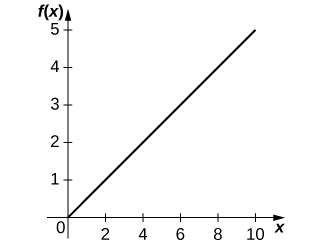b. $f^{\prime}(x)=a$. The more increase in prey, the more growth for predators.
c. $f''(x)=0$. As the amount of prey increases, the rate at which the predator population growth increases is constant.
d. This equation assumes that if there is more prey, the predator is able to increase consumption linearly. This assumption is unrealistic because we would expect there to be some saturation point at which there is too much prey for the predator to consume adequately.

23. [T] The Holling type II equation is described by $f(x)=\frac{ax}{n+x}$, where $x$ is the amount of prey available and $a < 0$ is the maximum consumption rate of the predator.

1. Graph the Holling type II equation given $a=0.5$ and $n=5$. What are the differences between the Holling type I and II equations?
2. Take the first derivative of the Holling type II equation and interpret the physical meaning of the derivative.
3. Show that $f(n)=\frac{1}{2}a$ and interpret the meaning of the parameter $n$.
4. Find and interpret the meaning of the second derivative. What makes the Holling type II function more realistic than the Holling type I function?

24. [T] The Holling type III equation is described by $f(x)=\frac{ax^2}{n^2+x^2}$, where $x$ is the amount of prey available and $a < 0$ is the maximum consumption rate of the predator.

1. Graph the Holling type III equation given $a=0.5$ and $n=5$. What are the differences between the Holling type II and III equations?
2. Take the first derivative of the Holling type III equation and interpret the physical meaning of the derivative.
3. Find and interpret the meaning of the second derivative (it may help to graph the second derivative).
4. What additional ecological phenomena does the Holling type III function describe compared with the Holling type II function?

#### Solution

a.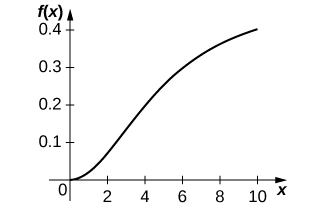b. $f^{\prime}(x)=\frac{2axn^2}{(n^2+x^2)^2}$. When the amount of prey increases, the predator growth increases.
c. $f''(x)=\frac{2an^2(n^2-3x^2)}{(n^2+x^2)^3}$. When the amount of prey is extremely small, the rate at which predator growth is increasing is increasing, but when the amount of prey reaches above a certain threshold, the rate at which predator growth is increasing begins to decrease.
d. At lower levels of prey, the prey is more easily able to avoid detection by the predator, so fewer prey individuals are consumed, resulting in less predator growth.

25. [T] The populations of the snowshoe hare (in thousands) and the lynx (in hundreds) collected over 7 years from 1937 to 1943 are shown in the following table. The snowshoe hare is the primary prey of the lynx.

Snowshoe Hare and Lynx Populations
Source: http://www.biotopics.co.uk/newgcse/predatorprey.html.
Population of snowshoe hare (thousands) Population of lynx (hundreds)
20 10
55 15
65 55
95 60
1. Graph the data points and determine which Holling-type function fits the data best.
2. Using the meanings of the parameters $a$ and $n$, determine values for those parameters by examining a graph of the data. Recall that $n$ measures what prey value results in the half-maximum of the predator value.
3. Plot the resulting Holling-type I, II, and III functions on top of the data. Was the result from part a. correct?

## Glossary

acceleration
is the rate of change of the velocity, that is, the derivative of velocity
amount of change
the amount of a function $f(x)$ over an interval $[x,x+h]$ is $f(x+h)-f(x)$
average rate of change
is a function $f(x)$ over an interval $[x,x+h]$ is $\frac{f(x+h)-f(a)}{b-a}$
marginal cost
is the derivative of the cost function, or the approximate cost of producing one more item
marginal revenue
is the derivative of the revenue function, or the approximate revenue obtained by selling one more item
marginal profit
is the derivative of the profit function, or the approximate profit obtained by producing and selling one more item
population growth rate
is the derivative of the population with respect to time
speed
is the absolute value of velocity, that is, $|v(t)|$ is the speed of an object at time $t$ whose velocity is given by $v(t)$